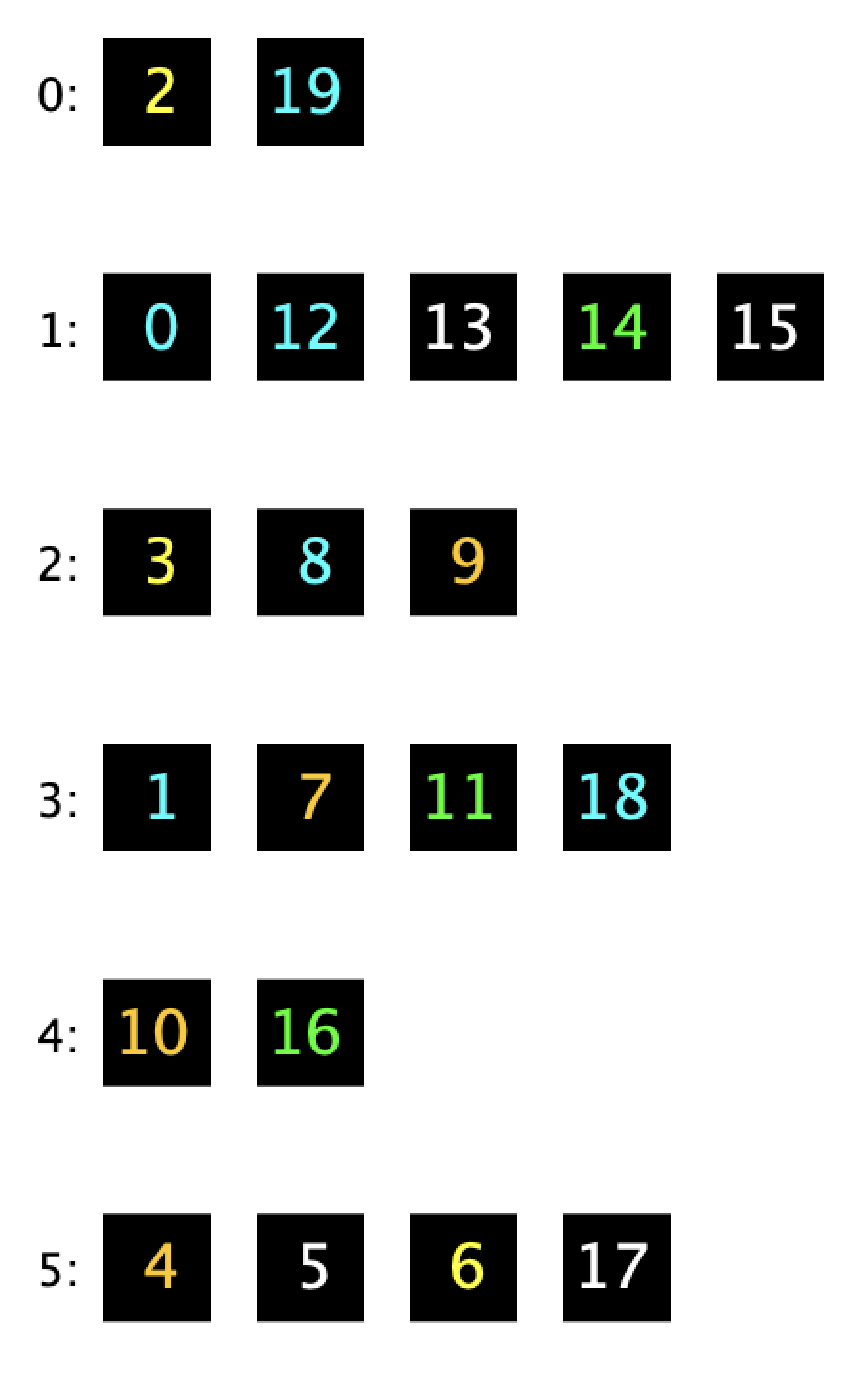Comment on page

# 20.3 Contains & Duplicate Items

## Contains

### The `equals()` Method for a ColoredNumber Object

Suppose the `equals()` method for ColoredNumber is as below, i.e. two ColoredNumbers are equal if they have the same num.
@Override
public boolean equals(Object o) {
if (o instanceof ColoredNumber otherCn) {
return this.num == otherCn.num;
}
return false;
}

### HashSet Behavior for Checking `contains()`

Suppose the `equals()` method for ColoredNumber is on the previous slide, i.e. two ColoredNumbers are equal if they have the same num.
int N = 20;
HashSet<ColoredNumber> hs = new HashSet<>();
for (int i = 0; i < N; i += 1) {
}
Suppose we now check whether 12 is in the hash table.
ColoredNumber twelve = new ColoredNumber(12);
hs.contains(twelve); //returns true
What do we expect to be returned by `contains`?
We expect the `contains` call to be true, all `12`s are created equal!

### Finding an Item Using the Default Hashcode

Suppose we are using the default hash function (uses memory address):
int N = 20;
HashSet<ColoredNumber> hs = new HashSet<>();
for (int i = 0; i < N; i += 1) {
}
ColoredNumber twelve = new ColoredNumber(12);
hs.contains(twelve); // returns ??
which yields the table below:Suppose equals returns true if two ColoredNumbers have the same num (as we've defined previously).
What is actually returned by `contains`?
Returns false with probability 5/6ths.
Default `hashCode()` is based on memory address. equals is based on `num`.
There are two ColoredNumber objects with `num = 12`. One of them is in the HashSet and one of them was created by the code above.
Each memory address is random, with only a 1/6th chance they modulo to the same bucket.
Example: If the `ColoredNumber` object `twelve` created by the code above is in memory location 6000000, its hashCode % 6 is 0. HashSet looks in bucket zero, and doesn't find 12.
Hard Question: If the default hash code achieves a good spread, why do we even bother to create custom hash functions?
It is necessary to have consistency between `equals()` and `hashCode()` for the hash table's operations to function.
Basic rule (also definition of deterministic property of a valid hashcode): If two objects are equal, they must have the same hash code so the hash table can find it.

## Duplicate Values

### Overriding `equals()` but Not `hashCode()`

Suppose we have the same `equals()` method (comparing `num`), but we do not override `hashCode()`.
public boolean equals(Object o) {
... return this.num == otherCn.num; ...
}
The result of adding 0 through 19 is shown below:ColoredNumber zero = new ColoredNumber(0);
hs.add(zero); // does another zero appear?
Which can happen when we call add(zero)?
1. 1.
We get a 0 to bin zero.
2. 2.
We add another 0 to bin one.
3. 3.
We add a 0 to some other bin.
4. 4.
We do not get a duplicate zero
3 Choices are Correct:
#1, #3, #4.
We get a 0 to bin zero, We add a 0 to some other bin, and we do not get a duplicate zero.
The new zero ends up in a random bin.
• 5/6ths chance: In bin 0, 2, 3, 4, or 5. Duplicate!
• 1/6 chance: In bin 1, no duplicate! (`equals()` blocks it)

## Key Takeaway: `equals()` and `hashCode()`

Bottom line: If your class override equals, you should also override hashCode in a consistent manner.
• If two objects are equal, they must always have the same hash code.
If you don’t, everything breaks:
• `Contains` can’t find objects (unless it gets lucky).
• `Add` results in duplicates.